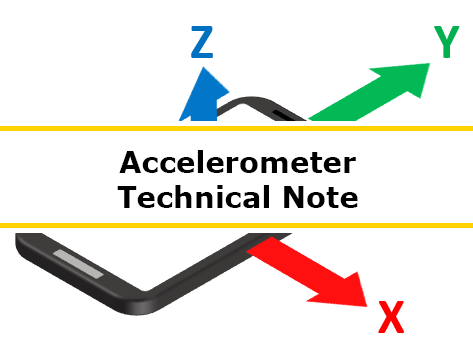# Accelerometer Technical Note

The following information is intended to supplement the Science Buddies tutorial on using the accelerometer sensor options in Google's Science Journal app. This resource provides more in-depth explanation of the science behind an accelerometer and the X, Y, Z, and Linear accelerometer options in the app.

## What is an accelerometer?

An accelerometer is a device that measures acceleration, which is the change in an object's velocity per second. Since velocity is measured in meters per second (m/s), acceleration is measured in meters per second per second, which is written as meters per second squared (m/s2). Technically, an accelerometer measures proper acceleration, which is not the same as coordinate acceleration. This means that the accelerometer can be used to detect the direction of gravity. This resource will explain what this means in more detail.

## How does the Science Journal app use the accelerometer?

Your phone has a built-in accelerometer, which Google's Science Journal app uses. To use the accelerometer in the app, you need to choose which accelerometer sensor you want to use based on what you are measuring and the direction the phone will be moving or tilting as part of the experiment. The Science Journal app includes separate sensors for Accelerometer X, Accelerometer Y, Accelerometer Z, and the Linear accelerometer. For more information about using the accelerometer sensors in the Science Journal app, see Exploring Acceleration with the Science Journal App.

## What does it mean that the accelerometer "detects gravity"? How can there be a non-zero acceleration reading even when the phone isn't moving?

Imagine holding a ruler by one end so that it is parallel to the ground. The ruler will bend slightly due to its own weight, as shown in Figure 1.:Figure 1.

Now imagine holding the ruler vertically up in the air (your hand at the bottom). At first, the ruler will be straight. But if you quickly move your hand to the side, the free end of the ruler will bend, as shown in Figure 2.:When a ruler is held vertically and gets pulled in a horizontal direction the point of the ruler furthest from the hand bends towards the opposite direction.

Figure 2.

In both of these cases, the ruler bends. In one case, it bends because of gravity (the ruler's own weight pulling it down). In the other case, it bends due to its change in velocity.

Now imagine dropping the ruler—holding it in front of you and letting go. In this case, the ruler will fall, but it will not bend at all.Figure 3.

Now imagine that you can somehow measure how much the ruler bends, but you do not have any other information about whether or not the ruler is moving. By looking only at the data, can you tell if the ruler bent because of the presence of gravity or because its velocity was changing? It turns out that you can't! Believe it or not, you just learned about the equivalence principal of Einstein's Theory of General Relativity. There's no way to tell (from the data alone) whether the ruler bent because of gravity or because its velocity was changing.

While their mechanical structure is a little more complicated than a plain ruler, accelerometers work exactly the same way. They are made up of parts that bend or deform when they accelerate and when gravity pulls on them. The 3-axis accelerometer in your phone has three of these structures arranged perpendicular to each other, allowing it to measure in the X, Y, and Z directions separately. Deformation of these structures is measured electrically. Based only on this electrical reading, there's no way to tell whether the deformation is because of the presence of gravity (Figure 1. above), because of changing velocity (Figure 2. above), or some combination of both. Technically this is called "proper acceleration" (as opposed to "coordinate acceleration," which is purely an object's change in velocity in a fixed coordinate system, not including any effects of gravity).

## But since gravity points down, shouldn't the Z accelerometer read negative 9.8 m/s2 when the phone is sitting face-up on a table?

Proper acceleration (discussed in the answer to the previous question) is defined as acceleration relative to an object in free-fall. Imagine that you have two phones: one sitting flat on a table and one that you drop (and pretend that you're able to drop it perfectly so it remains face-up). The phone that you drop will read zero acceleration in the Z direction (because it is in free fall, the "ruler" is not bending, similar to Figure 3 above). The phone that's on the table is sitting still, relative to you, but relative to the falling phone, it is accelerating "up" at a rate of 9.8 m/s2. Since Science Journal defines the positive Z direction as facing out of the phone's screen, the app will display positive 9.8 m/s2. If you flip the phone upside-down (so the negative Z direction points "up"), then it will display negative 9.8 m/s2. (To see this in action, see the sample graph in the Exploring Acceleration with the Science Journal App tutorial.)

## If gravity affects the accelerometer readings, then how does the linear accelerometer report acceleration without including gravity?

Above, we explained that if you don't have any other information, then it is impossible to tell the difference between gravity and a change in velocity using an accelerometer. However, your phone has a trick up its sleeve. It also contains a magnetometer, a device that measures magnetic fields. The magnetometer helps the phone figure out which way is "down" by first figuring out which way Earth's magnetic field is pointing. Once the phone knows which way is down, it can subtract the effect of gravity from the accelerometer reading. This is why the linear accelerometer reads 0 m/s2 when you put the phone flat on a table. Even though there is an acceleration of 9.8 m/s2 in the Z direction, the phone knows that the Z axis is pointing up (perpendicular to the ground), so it subtracts that value before calculating the linear acceleration.

## Why are linear accelerometer values never negative?

You might find it confusing that the linear accelerometer values always stay positive, even when the phone "slows down" or changes direction. Shouldn't the acceleration be negative when that happens? The short answer is that the linear acceleration is the magnitude of the combined X, Y, and Z accelerations, excluding gravity. Acceleration is a vector, which is like an arrow in space that goes from one point to another. A vector has both a magnitude (a length) and a direction. The magnitude of the vector is always positive, regardless of which way the vector points (just like how an object's length is always positive, regardless of how you rotate the object). If that explanation satisfies you, great! If not, keep reading to learn a little more about vectors.

When we draw vectors, we represent them with arrows. This vector goes from point A to point B. To make things easier to write, we can just call it "vector AB":Figure 4.

Two or more vectors can be combined to make one equivalent vector. For example, here the vector that goes from A to B (vector AB) can be combined with vector BC, to form the equivalent vector AC: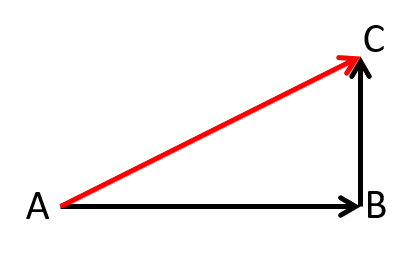Figure 5.

The X, Y, and Z accelerations are all vectors that are defined along a specific axis. Those axes have clearly defined positive and negative directions. For example, when you look at your phone's screen, positive Y is up, negative Y is down, positive X is to the right, and negative X is to the left. Individually, these values can be either positive or negative (where "positive" means that the vector points in the positive direction, and "negative" means that the vector points in the negative direction). For example, the red and green arrows here show an X acceleration of +4 m/s2 and a Y acceleration of -3 m/s2 respectively: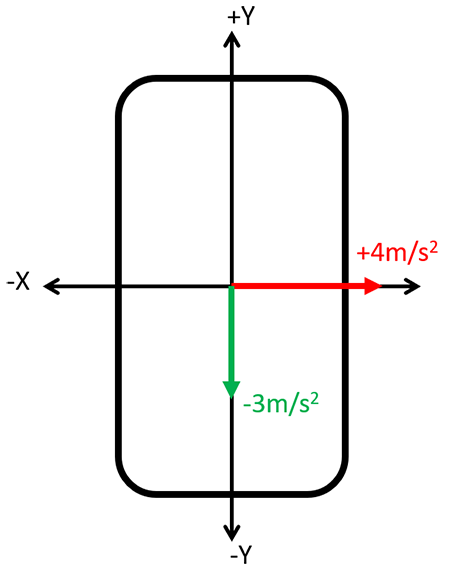Figure 6.

Those two acceleration vectors can be combined by connecting them end to end (like in Figure 5), to form one equivalent acceleration vector, shown in purple. (Note: we're leaving Z acceleration out to keep these drawings two-dimensional and easier to follow):Figure 7.

However, there's no way to say whether that vector is "positive" or "negative." It points along the positive X axis, but the negative Y axis. We can still measure the length of the vector, which is always positive, regardless of which way it is pointing. This is why the linear acceleration values are always positive—the linear accelerometer is measuring the length of the combined X, Y, and Z acceleration vectors (after subtracting out gravity, which is also a vector).

You can also explain this using a little bit of math. Does the drawing of vectors AB, BC, and AC (shown in Figure 5.) look familiar? If you've learned about the Pythagorean Theorem in school, you should recognize it as a right triangle. If you know the lengths of any two sides of the triangle, you can calculate the length of the third side using this equation:

You can rearrange that equation to solve for the length of the hypotenuse (the longest side) if you know the lengths of the other two sides:

Since the square of a real number is always positive, then according to this equation, c will always be positive, even if a or b (or both) are negative. The same concept applies in three dimensions for the X, Y, and Z accelerations.

## Does a positive acceleration value always mean the phone is "speeding up"? Does a negative acceleration value always mean the phone is "slowing down"?

The short answer is "not necessarily." When interpreting data from the accelerometer, you have to be careful with phrases like "speeding up" and "slowing down," or words like "accelerate" and "decelerate." First, as described in the previous question, the linear acceleration value is always positive, even if the phone is "slowing down." And as described earlier, depending on the phone's orientation, the X, Y, and Z accelerometers can have a non-zero acceleration reading due to gravity, even if the phone's velocity is not changing. However, even when using the X, Y, and Z accelerometers and carefully controlling the phone's orientation, you have to be careful about the sign convention (the definition of which way is positive and which way is negative) for acceleration and how you describe it.

To show this, put your phone flat on the floor and open the Accelerometer Y sensor. Start a recording, and then give your phone a shove in the positive Y direction. Let it slide until it comes to a stop. Then stop the recording. You should see something like this: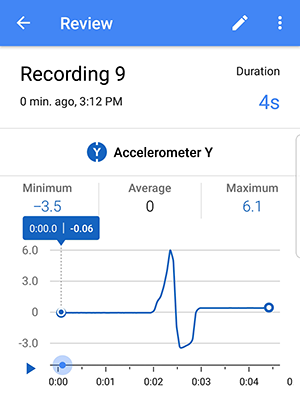Figure 8.

In the first part of the graph, there is a positive acceleration when you push the phone. During this time, its velocity increases. In everyday language we'd say that it "accelerates" or "speeds up." In the second part of the graph, there is a negative acceleration as the phone slides to a stop. In everyday language we'd say that it "decelerates" or "slows down."

Now try the same thing, but this time, flip the phone around so you push it in the negative Y direction. Your graph should look something like this: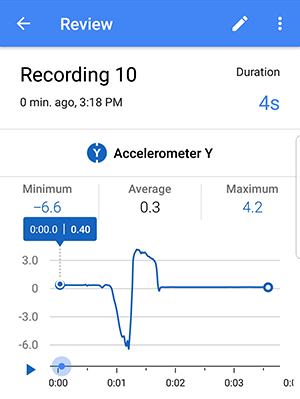Figure 9.

This time, the graph is reversed. First there is a negative acceleration when you push the phone, and then a positive acceleration when the phone coasts to a stop. However, even though it has a negative acceleration, you wouldn't say that the phone is "decelerating" or "slowing down" when you push it, or, conversely, even though it has a positive acceleration, that it's "accelerating" or "speeding up" when it coasts to a stop. Suddenly, the everyday language we use when referring to speed and acceleration becomes confusing. If you assume that "negative acceleration" means "slowing down," then your interpretation of the graph could be wrong.

The problem arises because of an important distinction between the words speed and velocity. In everyday language, we often use them interchangeably. However, in physics, velocity is a vector, and speed is the magnitude of that vector (review the section about vectors if this isn't clear). When talking about velocity in only one direction, that means the velocity can be positive or negative, but speed is always positive (in other words, the speed is the absolute value of the velocity). When we say something is "accelerating" or "speeding up," we generally mean that its speed is increasing, or that the velocity is getting farther away from zero (either in the positive or negative direction). This meaning is clear when the velocity is getting "more positive" (e.g. changing from +10 m/s to +15 m/s) because the acceleration, or change in velocity, is positive.

However, it gets confusing when the velocity is getting "more negative" (e.g. changing from -10 m/s to -15 m/s). While the object's velocity is decreasing, the speed (absolute value of the velocity) is increasing. So, even though the acceleration is negative, you could still say that the object is "speeding up." Conversely, when an object's velocity is becoming "less negative" (e.g. changing from -15 m/s to -10 m/s), it has a positive acceleration, but its speed is decreasing, so you would say that it is "slowing down."

So, be careful when interpreting acceleration graphs from your experiments and make sure you account for which direction your phone was pointing! You will need to be extra careful when doing any experiment in which the phone moves back and forth (e.g., is attached to a pendulum, an oscillating spring, or a moving person), since the phone's velocity and acceleration will both change from positive to negative.